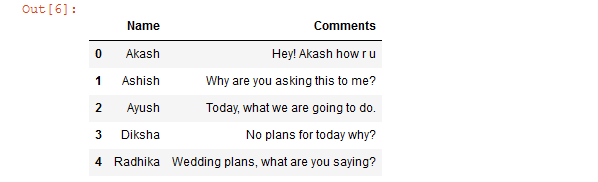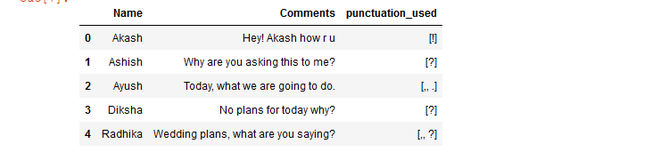# Extract punctuation from the specified column of Dataframe using Regex

• Last Updated : 29 Dec, 2020

Prerequisite: Regular Expression in Python

In this article, we will see how to extract punctuation used in the specified column of the Dataframe using Regex.

Firstly, we are making regular expression that contains all the punctuation: [!”\\$%&\'()*+,\-.\/:;=#@?\[\\\]^_`{|}~]* Then we are passing each row of specific column to re.findall() function for extracting the punctuation and then assigning that extracted punctuation to a new column in a Dataframe.

re.findall() function is used to extract all non-overlapping matches of pattern in string, as a list of strings. The string is scanned left-to-right, and matches are returned in the order found.

Syntax: re.findall(regex, string)

Return: All non-overlapping matches of pattern in string, as a list of strings.

Now, Let’s create a Dataframe:

## Python3

 `# import required libraries``import` `pandas as pd``import` `re`` ` `# creating Dataframe with``# name and their comments``df ``=` `pd.DataFrame({``    ``'Name'` `: [``'Akash'``, ``'Ashish'``, ``'Ayush'``,``              ``'Diksha'` `, ``'Radhika'``],``   ` `    ``'Comments'``: [``'Hey! Akash how r u'` `, ``                 ``'Why are you asking this to me?'` `,``                 ``'Today, what we are going to do.'` `,``                 ``'No plans for today why?'` `,``                 ``'Wedding plans, what are you saying?'``]},``   ` `    ``columns ``=` `[``'Name'``, ``'Comments'``]``    ``)`` ` `# show the Dataframe``df`

Output:Now, Extracting the punctuation from the column comment:

## Python3

 `# define a function for extracting``# the punctuations``def` `check_find_punctuations(text):``   ` `    ``# regular expression containing``    ``# all punctuation``    ``result ``=` `re.findall(r``'[!"\\$%&\'()*+,\-.\/:;=#@?\[\\\]^_`{|}~]*'``, ``                        ``text)``     ` `    ``# form a string``    ``string ``=` `"".join(result)``     ` `    ``# list of strings return``    ``return` `list``(string)``   ` `# creating new column name``# as a punctuation_used and ``# applying user defined function``# on each rows of Comments column``df[``'punctuation_used'``] ``=` `df[``'Comments'``].``apply``(``                         ``lambda` `x : check_find_punctuations(x)``                         ``)`` ` `# show the Dataframe``df`

Output:Attention geek! Strengthen your foundations with the Python Programming Foundation Course and learn the basics.

To begin with, your interview preparations Enhance your Data Structures concepts with the Python DS Course. And to begin with your Machine Learning Journey, join the Machine Learning – Basic Level Course

My Personal Notes arrow_drop_up